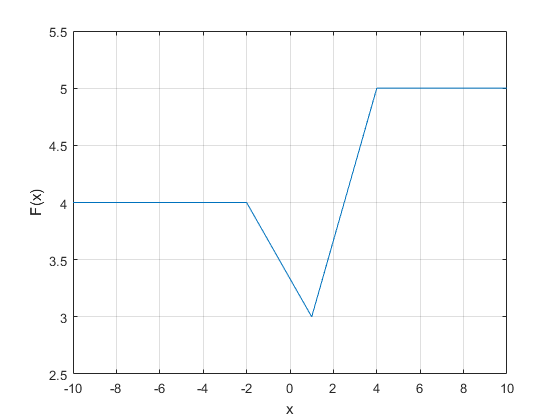# pwlinear

Create a piecewise-linear nonlinearity estimator object

## Syntax

```NL = pwlinear NL = pwlinear(Name,Value) ```

## Description

`NL = pwlinear` creates a default piecewise-linear nonlinearity estimator object with 10 break points for estimating Hammerstein-Wiener models. The value of the nonlinearity at the break points are set to `[]`. The initial value of the nonlinearity is determined from the estimation data range during estimation using `nlhw`. Use dot notation to customize the object properties, if needed.

`NL = pwlinear(Name,Value)` creates a piecewise-linear nonlinearity estimator object with properties specified by one or more `Name,Value` pair arguments. The properties that you do not specify retain their default value.

## Object Description

`pwlinear` is an object that stores the piecewise-linear nonlinearity estimator for estimating Hammerstein-Wiener models.

Use `pwlinear` to define a nonlinear function $y=F\left(x,\theta \right)$, where y and x are scalars, and θ represents the parameters specifying the number of break points and the value of nonlinearity at the break points.

The nonlinearity function, F, is a piecewise-linear (affine) function of x. There are `n` breakpoints (xk,yk), k = 1,...,n, such that yk = F(xk). F is linearly interpolated between the breakpoints.

F is also linear to the left and right of the extreme breakpoints. The slope of these extensions is a function of xi and yi breakpoints. The breakpoints are ordered by ascending `x`-values, which is important when you set a specific breakpoint to a different value.

There are minor difference between the breakpoint values you set and the values stored in the object because the toolbox has a different internal representation of breakpoints.

For example, in the following plot, the breakpoints are xk = [-2,1,4] and the corresponding nonlinearity values are yk = [4,3,5].The value `F(x)` is computed by `evaluate(NL,x)`, where `NL` is the `pwlinear` object. When using `evaluate`, the break points have to be initialized manually.

For `pwlinear` object properties, see Properties.

## Examples

collapse all

`NL = pwlinear;`

Specify the number of break points.

`NL.NumberOfUnits = 5;`

```load twotankdata; z = iddata(y,u,0.2,'Name','Two tank system'); z1 = z(1:1000);```

Create a `pwlinear` object, and specify the breakpoints.

`InputNL = pwlinear('BreakPoints',[-2,1,4]);`

Since `BreakPoints` is specified as a vector, the specified vector is interpreted as the x-values of the break points. The y-values of the break points are set to 0, and are determined during model estimation.

Estimate model with no output nonlinearity.

`sys = nlhw(z1,[2 3 0],InputNL,[]);`

## Input Arguments

### Name-Value Pair Arguments

Specify optional comma-separated pairs of `Name,Value` arguments. `Name` is the argument name and `Value` is the corresponding value. `Name` must appear inside quotes. You can specify several name and value pair arguments in any order as `Name1,Value1,...,NameN,ValueN`.

Use `Name,Value` arguments to specify additional properties of `pwlinear` nonlinearity. For example, `NL= pwlinear('NumberofUnits',5)` creates a piecewise-linear nonlinearity estimator object with 5 breakpoints.

## Properties

`pwlinear` object properties include:

 `NumberofUnits` Number of breakpoints, specified as an integer. Default: `10`
 `BreakPoints` Break points, xk, and the corresponding nonlinearity values at the breakpoints, yk, specified as one of the following: 2-by-n matrix — The x and y values for each of the n break points are specified as [x1,x2, ...., xn;y1, y2, ..., yn].1-by-n vector — The specified vector is interpreted as the x values of the break points: x1,x2, ...., xn. All the y values of the break points are set to `0`. When the nonlinearity object is created, the breakpoints are ordered by ascending `x`-values. This is important to consider if you set a specific breakpoint to a different value after creating the object. Default: `[]`

## Output Arguments

collapse all

Piecewise-linear nonlinearity estimator object, returned as a `pwlinear` object.